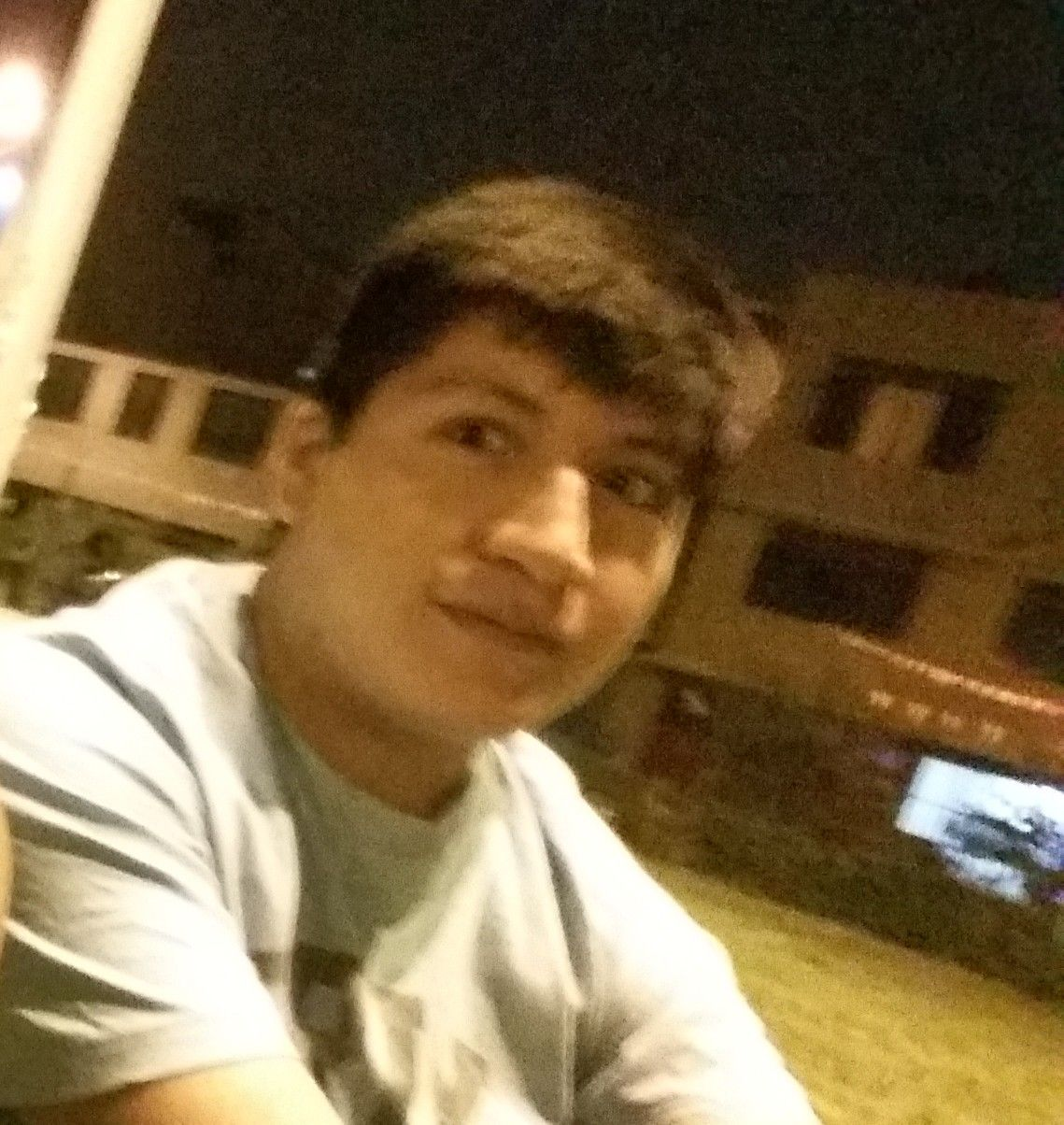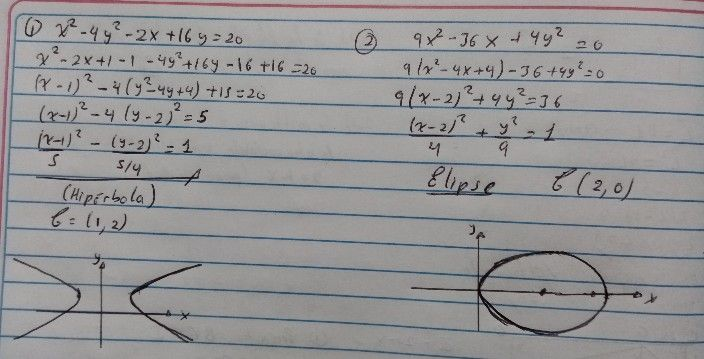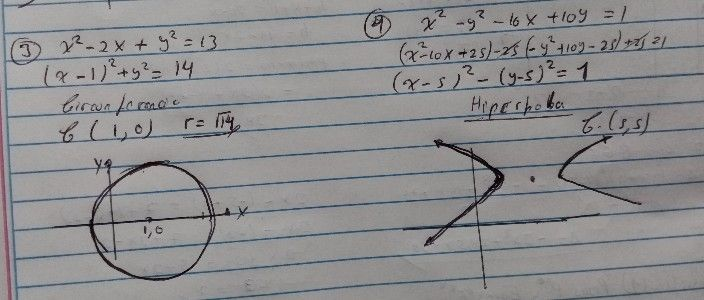Símbolos
Problema$1:$ $x^{2}-4y^{2-2x+\right)dy}=20$ $2:$ $9x^{2}-3\left(\times$ $+y_{y}2=0$ $3=x^{2}-2x$ $1y^{2}=13$ $y\div$ $x^{2}-y^{2}$ $y^{2}$ $=$ $10$ $\left(x-y\right)+1$
Bachillerato
Trigonometría
Búsquedas: 119
SoluciónProfesor de Qanda - Luis